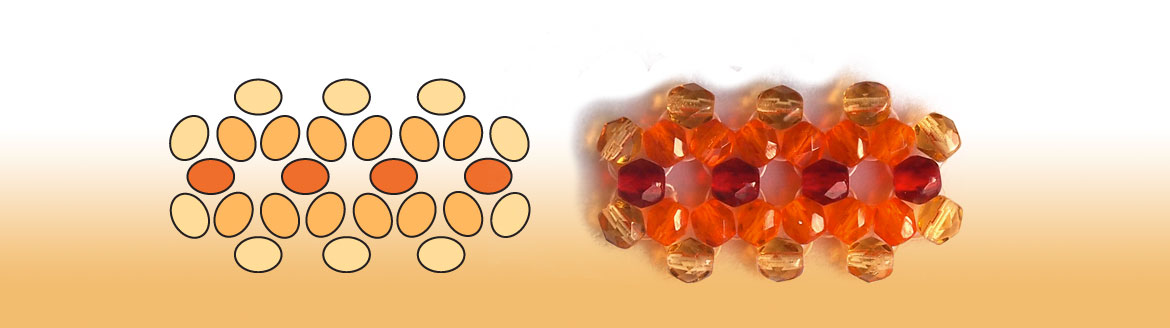Posted on

# Triangle weave lesson 2 – free beading lesson for beginnersIn this lesson you can learn, how to make a U-turn with triangle weave, and how to make a dual row triangle weave chain.

### Content

• TRIANGLE FORMS
• How can you improve your triangles?
• DUAL ROW TRIANGLE WEAVE CHAIN
• U-turn with triangle weave
• How to make a U-turn with triangle weave?
• The second triangle weave row
• How to Make a dual row triangle weave chain?

You can find theTriangle weave lesson 2 here >>